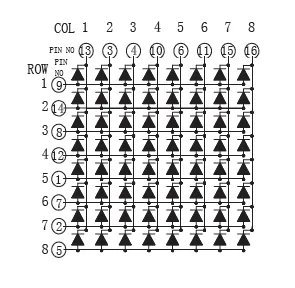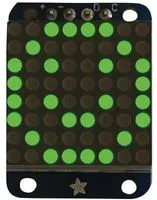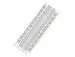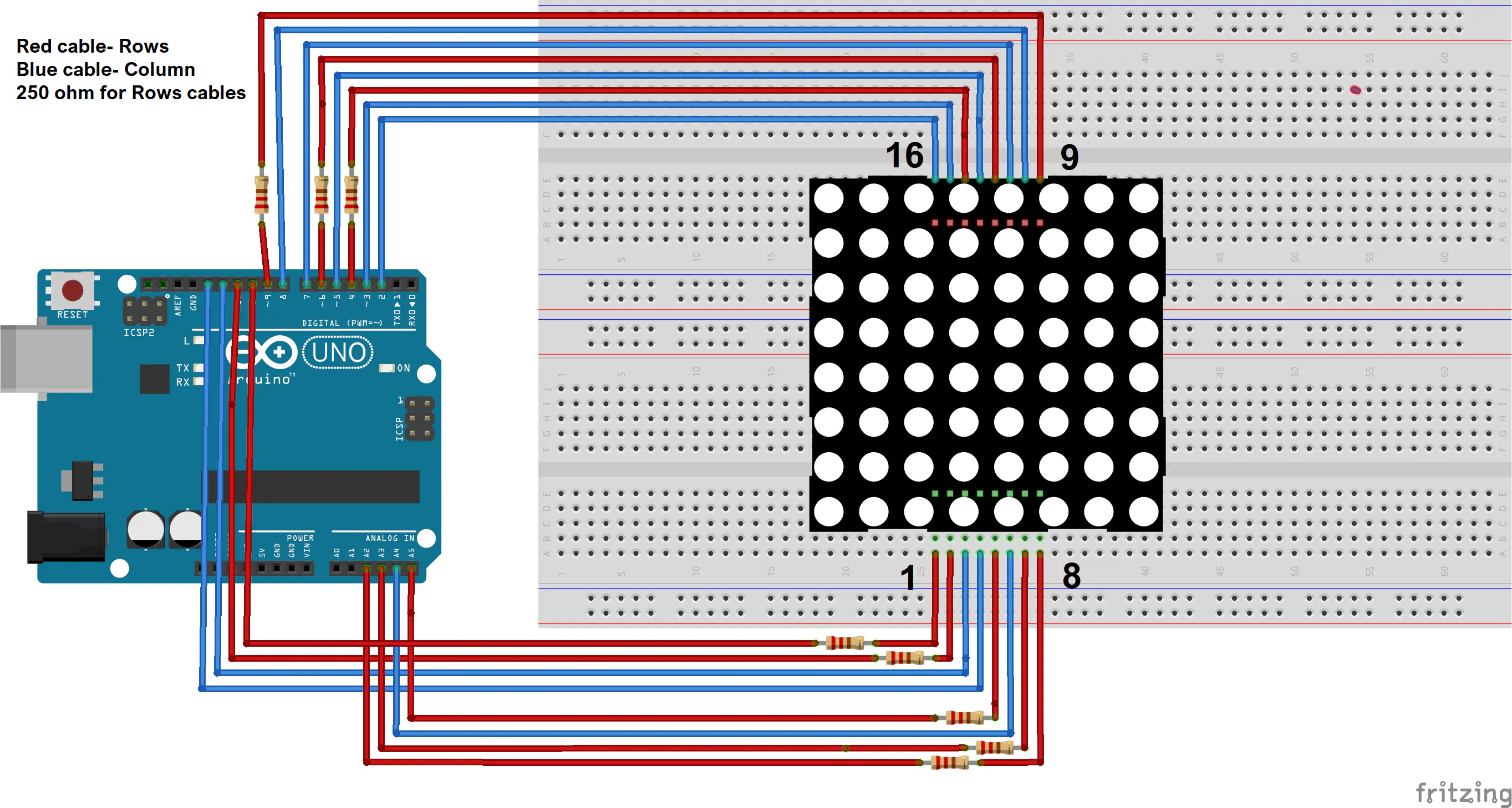# 8x8 led matrix interfacing with arduino

I use 788bs, a common anode 8x8 led matrix Modules for this project. Learn how to identify matrix pinout, how to interface with Arduino.

IntermediateFull instructions provided59,761## Things used in this project

### Hardware componentsArduino UNO
×1LED Dot Matrix Display, Green
×1×1AA Batteries
×1Jumper wires (generic)
×1

### Software apps and online servicesArduino IDE

### Hand tools and fabrication machinesDigilent Mastech MS8217 Autorange Digital MultimeterMultitool, Screwdriver

## Schematics

### Pin connection led matrix

8x8 led matrix connection diagram## Code

### Led matrix display running

INI
To display as you like, I display a moving a Person.
```// Pins connect as per define
// Fill in the pins you used on your own setup.

#define ROW_1 2
#define ROW_2 3
#define ROW_3 4
#define ROW_4 5
#define ROW_5 6
#define ROW_6 7
#define ROW_7 8
#define ROW_8 9

#define COL_1 10
#define COL_2 11
#define COL_3 12
#define COL_4 13
#define COL_5 A0
#define COL_6 A1
#define COL_7 A2
#define COL_8 A3

const byte rows[] = {
ROW_1, ROW_2, ROW_3, ROW_4, ROW_5, ROW_6, ROW_7, ROW_8
};

// The display buffer
// It shows a walking steps (1 = ON, 0 = OFF)
byte star1[] = {B00000110,B11000010,B10100100,B00011000,B00011000,B00100101,B01000011,B01100000};
byte star2[] = {B00110000,B00100000,B00100111,B00011001,B10011000,B11100100,B00000100,B00001100};
byte star3[] = {B00000110,B10001000,B10010000,B01011100,B00111010,B00001001,B00010001,B01100000};

float timeCount = 0;

void setup() {
// Open serial port
Serial.begin(9600);

// Set all used pins to OUTPUT
// This is very important! If the pins are set to input
for (byte i = 2; i <= 13; i++)
pinMode(i, OUTPUT);
pinMode(A0, OUTPUT);
pinMode(A1, OUTPUT);
pinMode(A2, OUTPUT);
pinMode(A3, OUTPUT);
}

void loop() {
// This could be rewritten to not use a delay, which would make it appear brighter
delay(5);
timeCount += 1;
if(timeCount <  20) {
drawScreen(star1);
} else if (timeCount <  80) {
drawScreen(star2);
} else if (timeCount <  100) {
drawScreen(star3);
} else if (timeCount <  120) {
drawScreen(star1);
} else if (timeCount <  140) {
drawScreen(star2);
} else if (timeCount <  160) {
drawScreen(star3);
} else {
// back to the start
timeCount = 0;
}
}
void  drawScreen(byte buffer2[]){

// Turn on each row in series
for (byte i = 0; i < 8; i++) {
setColumns(buffer2[i]); // Set columns for this specific row

digitalWrite(rows[i], HIGH);
delay(2); // Set this to 50 or 100 if you want to see the multiplexing effect!
digitalWrite(rows[i], LOW);

}
}

void setColumns(byte b) {
digitalWrite(COL_1, (~b >> 0) & 0x01); // Get the 1st bit: 10000000
digitalWrite(COL_2, (~b >> 1) & 0x01); // Get the 2nd bit: 01000000
digitalWrite(COL_3, (~b >> 2) & 0x01); // Get the 3rd bit: 00100000
digitalWrite(COL_4, (~b >> 3) & 0x01); // Get the 4th bit: 00010000
digitalWrite(COL_5, (~b >> 4) & 0x01); // Get the 5th bit: 00001000
digitalWrite(COL_6, (~b >> 5) & 0x01); // Get the 6th bit: 00000100
digitalWrite(COL_7, (~b >> 6) & 0x01); // Get the 7th bit: 00000010
digitalWrite(COL_8, (~b >> 7) & 0x01); // Get the 8th bit: 00000001

// If the polarity of your matrix is the opposite of mine
// remove all the '~' above.
}
```

## Credits

### Shahbaz75sb

0 projects • 1 follower